### the Detection of Facial Features

Michael MCGuffin

University of Toronto

submitted June 2002

A course project completed for CSC 2503: Computational Vision I (Prof. Allan Jepson)

### Summary

As a first step toward building a face detector, two ad hoc strategies were explored. The first attempts to detect lines of symmetry in subsets of an image, as a way of guessing where a human face might be. The second tries to find the centres of loops of edgels that may correspond to eyes. The implementation of these two strategies is described, results of testing are given, and methods for tweaking and improving the algorithms are given. The integration of these two strategies into a more complete face detector is also discussed.

### Motivation

There were two main motivations for this project. First, a fast face detector could be used for research into Perceptual User Interfaces (PUIs). For example, a PUI could be constructed where the computer can "see" the user through a camera and respond to head motion or even changes in facial expression. Interesting experiments could be performed even if the detector is approximate, and uses hard-coded assumptions (e.g. expecting to only ever see one face, to see it approximately facing forward and upright, to always see it at approximately the same size, etc.)

Secondly, although fairly robust detectors already exist (some of which may even be freely available for downloading), I wanted to gain implementation experience, and also try out some of my own naive ideas about how to perform face detection.

### Related Work

Cootes et al.  describe Active Shape Models (ASMs) and Active Appearance Models (AAMs) that can be matched to the features in an image of a face. Both types of models are built from a set of training images. ASMs match a model to boundaries (e.g. edges) in an image. AAMs find model parameters that reproduce the texture of the image as closely as possible. Both techniques seem to assume that a face is present in the image to be matched, and seem to require approximate initialization to allow convergence upon a match.

Herpers et al.  describe GAZE, a system for detecting and analyzing faces using an "attentional mechanism" inspired by eye movements in humans. For example, if a right eye is identified ("foveated") in the input image, there is the expectation that another eye will appear to the left of the right eye, and attention is thus temporarily increased in that region to help detect the second eye.

Even more ambitious work has moved toward extracting and interpreting facial expressions [1, 2, 3]. The extraction of this information has the potential to make PUIs even more useful, since it adds many degrees of freedom for input.

### Approach

Two algorithms were implemented: one for detecting symmetry, and one for detecting eyes.

Symmetry Detection

If a region of an image contains a forward-facing human face, we can reasonably expect there to be a line of symmetry along the major axis of the face that approximately reflects one half of the face onto the other. Rather than search for symmetry among the raw pixel data, I propose searching for lines of symmetry between edgels, since there are usually many fewer of these than raw pixels (not to mention that, when a face is lit from the side or is in partial shadow, the symmetry in the raw image probably suffers more than the symmetry present in edgel data).

To do this, we can first detect all the edgels in the input image, then search for pairs of cocircular edgels (i.e. edgels whose relative positions and gradient orientations are such that a line of symmetry exists between them), and then search for common lines of symmetry that are suggested by many pairs of cocircular edgels.

Unfortunately, an image containing E edgels will contain O(E2) pairs of cocircular edgels, and this can considerably slow down searching. Strategies for cutting down this number will be discussed in the Future Directions section.

Eye Detection

The shading around an eye often gives rise to a "loop" or "chain" of edgels surrounding the eye. Although the loop may not be strictly closed, it is typically nearly closed. One approach for detecting these loops is to define an objective function over the image space such that loops of edgels will create a local maximum at the centre of the loop. Given such an objective function, we can then search for local maxima within it.

The objective function could be a summation of components, where each edgel contributes one component. For example, if we know the expected radius reye of eyes, and we expect eyes to be darker than the skin surrounding the eyes, then we can expect most of the edgels surrounding an eye to have gradients pointing outward. Since we would like the objective function to have a maximum at the centre of the eye, we could define the contribution of each edgel to be a small positive "bump" (i.e. a central maximum with a surrounding falloff) at a distance reye from the edgel and in the direction opposite the edgel's gradient. This way, if many edgels are arranged radially around a centre c and at a distance reye, and if most of the edgel's gradients point away from c, then the components should add up to a large local maximum near c (Figure 1).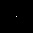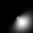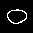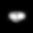Figure 1. (Upper left) An isolated edgel, whose gradient points toward the upper left at a 45 degree angle. (Upper right) The edgel's contribution to the objective function, where reye = 8 pixels. (Lower left) A loop of edgels, whose gradients point outward. The radius of the loop is approximately 8 pixels. (Lower right) The resulting objective function, after rescaling -- note the maximum within the loop (actually it looks like two maxima in this case, but this is approximately what is desired).

Unfortunately, it may happen that the eye (e.g. the interior of the loop) is lighter than the surrounding pixels. In this case, we would have to flip the direction in which edgels contribute a bump component to the objective function. Fortunately, we can overcome this problem by simply having each edgel contribute two bumps: one in each direction, and each at a distance of reye. This way, the bumps in the centre of a loop will add up to a large local maximum, which should be easy to search for, despite the possibility of a surrounding "ring" of smaller local maxima (Figure 2).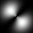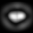Figure 2. (Upper left) An isolated edgel, whose gradient points toward the upper left at a 45 degree angle. (Upper right) The edgel's contribution to the objective function. (Lower left) A loop of edgels, whose gradients point outward. (Lower right) The resulting objective function, after rescaling -- note the maximum (or, actually, 2 maxima) within the loop and also the surrounding ring of smaller maxima. Contrary to Figure 1, the objective function here is invariant under global inversions of edgel gradients. Hence, we could invert the grey levels in the input image, and still get the same objective function.

Essentially, the scheme presented here for detecting eyes amounts to a way of filtering the edgel data to try and amplify loop centres.

### Implementation

The software for this project was written from scratch in C++ on linux. About 1500 lines of code were written in total. OpenGL and GLUT were used for displaying the input image and the output of the various algorithms.

Input images were stored as PGM (portable grey map) files , and a routine for reading in PGM files was implemented. A routine was also written for performing Canny edgel detection, using the CSC 2503 Matlab routines  as a guide.

Symmetry Detection

Below (Figure 3) is an example input image with the resulting edgel information computed from it.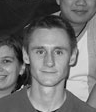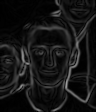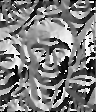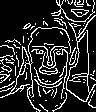Figure 3. From left to right: an input image (96x112 pixels), the gradient magnitude image, the gradient orientation image, and the edgel presence image after non-max suppression (1812 edgels detected).

From the edgel image (Figure 3, right-most image) we generate a list of edgels. Let E be the number of edgels. We then check all E(E-1)/2 pairs of edgels, testing each pair for cocircularity (i.e. to see if there is a line of symmetry between the edgels that reflects one onto the other). Since we expect some noise to be present in the input image, and we also want to allow for slight deviations from perfect symmetry (as would occur if a face is turned slightly away from the camera), the cocircularity test allows for a mismatch of +/- thetaepsilon = 0.1 radians in the edgel orientations.

To cut down slightly on the number of cocircular pairs that need to be considered later, the cocircularity check also rejects any pair of edgels that are too far apart or too close (i.e. if the distance between the edgels falls outside of [Dmin*W,Dmax*W] = [0.05*W,0.8*W], where W is the width of the input image, then the pair is rejected).

For each pair of edgels that passes the cocircularity test, we compute the line of symmetry between them, and then add this line to a list of candidate lines of symmetry. Lines of symmetry are described by parameters a1 and a2, where the vector equation of the line is (cos(a1),sin(a1))*x+a2==0. The parameter space a1-a2 is a dual space with respect to the image space: lines in image space correspond to points in parameter space, and multiple lines in image space that intersect at a common point will correspond to colinear points in parameter space -- hence we can define a mapping from lines in parameter space to points in image space.

The software implemented actually plots all the candidate lines of symmetry found in parameter space (essentially performing a Hough transform) to help visualize the functioning of the algorithm and confirm that it is working.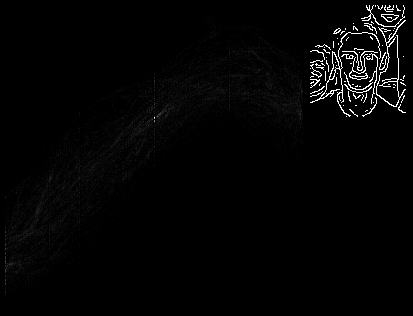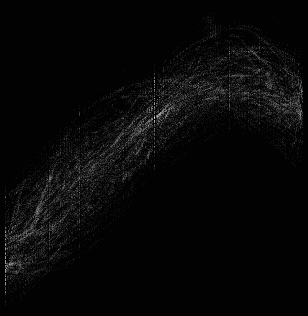Figure 4. (Left) A plot of candidate lines of symmetry in parameter space (the horizontal axis is a1, and the vertical is a2), and the edgel data used to find candidates lines of symmetry. Note the single bright spot in parameter space: this corresponds to a line of symmetry that is shared by many pairs of cocircular edgels. (Right) The same plot of parameter space, but with grey levels adjusted to increase brightness.

Furthermore, the user can select a point in parameter space by pointing the mouse cursor at it, and see the corresponding line of symmetry drawn in image space. In this way, the user can interactively explore the parameter space and get a feel for the distribution of cocircular edgel pairs.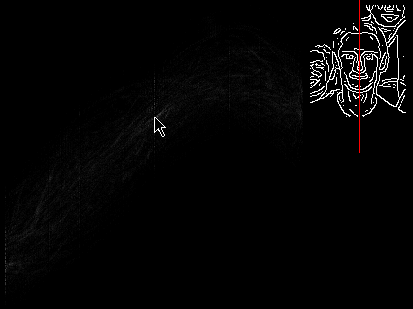Figure 5. The mouse cursor placed over the bright spot in parameter space, and the corresponding line of symmetry drawn in red over the edgel data.

It is possible to search through the parameter space and automatically find lines of symmetry shared by many pairs of edgels, using (for example) M-estimation. M-estimation would involve choosing a candidate line of symmetry as an initial guess, and iteratively computing a new guess by summing together all candidates weighted by (for example) a Cauchy estimator, and continuing this until we converge on a line of symmetry shared by many pairs of edgels. This could be repeated many times to detect multiple lines of symmetry.

In fact, a brief, preliminary investigation into using M-estimation was performed. Although time restrictions prevented the implementation from being properly tested and completed, initial tests with it seemed to indicate that convergence was very sensitive to the choice of initial estimate. Randomly chosen samples didn't seem to work well as initial estimates, as they prevented convergence on large maxima. Hence, efforts were refocused on finding maxima in the Hough transform, since the parameter space is only 2-dimensional, and maxima found in the Hough transform could serve as good initial estimates for M-estimation, which itself could be completed at a later date.

Note that it is not immediately clear how to find maxima in the Hough transform, since multiple faces in the input image should lead to multiple local maxima in the Hough transform, however other "near symmetries" in the edgel data can lead to smaller local maxima. To aid in the development of a proper algorithm for finding maxima, a routine for printing out a histogram of pixel values in the Hough transform image was implemented.

This lead to the development of an "adaptive" thresholding technique to find maxima. The minimum threshold tau0 is computed such that no more than 0.01 % of the pixels in the Hough transform image exceed its value. Then, a higher threshold tau = (1+tau0)/2 is used to select the largest pixel values in the Hough transform. Finally, non-maximum suppression is applied to further eliminate pixels. The lines of symmetry corresponding to the remaining pixels are the final output of the symmetry detector.

Eye Detection

To perform loop detection, an image of the objective function is generated. This is equivalent to filtering the edgel image with the filter in Figure 2 (upper right), and an example of the result is show in Figure 6.

Next, adaptive thresholding is performed (as described for symmetry detection), and non-max suppression is applied. The resulting maxima is the final output of the loop detector (Figure 6).

Note that the expected radius reye of eyes must be manually tuned, however in practice it can be inaccurate by a few pixels and still detect the centres of eyes (see Results section).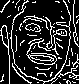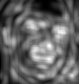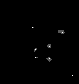Figure 6. From left to right: an input image, the edgel image, the objective function image, and the objective function image after thresholding (grey pixels are non-maxima, white pixels are maxima). Note the two maxima corresponding to the eyes. For the computation of the objective function here, reye = 4 pixels.

### Results

The system was tested with photos of people, photos of dolls, paintings, and drawings, each containing either one or more faces at different orientations and with different environmental surroundings. Unfortunately, the brute-force nature of the symmetry detector made it impractical to test very large images.

In all the test images below, reye = 4 pixels. Although this is probably not the optimal value for many of the test images, it is informative (and probably more realistic) to see how well the system performs even with reye set to a slightly inaccurate value.

A summary of the results is shown in the below table. The last two columns give a rough "score" of how well the algorithms performed on each test image.

The symmetry detector worked well in some cases, and very poorly in others (e.g. Figure 11).

There were some cases (e.g. Figures 12 and 13) where the symmetries of the faces were not detected, however interactive exploration of the Hough transform revealed that there were local maxima (bright spots) corresponding to the true symmetries of the faces. Unfortunately, these local maxima were rejected by the adaptive thresholding.

A similar problem is shown in Figure 15: in the 2nd image, we see the threshold is too high to detect all three lines of symmetry of the faces. Unfortunately, lowering the threshold enough to detect all three lines (shown in the 3rd image) means that many undesired lines of symmetry are also detected.

Notice that in some cases, the symmetry detector seems to find lines reflecting one face onto another (e.g. Figures 12, 13, 15).

The symmetry detector's brute force search made it very slow for some images: Figure 11 required many minutes (on the order of 20-30) of CPU time on a 600 MHz K7 processor.

The loop detector worked well in many cases, even though reye = 4 pixels was not optimal for all images (e.g. Figure 10). There is the potential, however, for it to identify many loops in the image which do not correspond to eyes (e.g. Figure 11 shows an example where there were many maxima in the objective function that did not correspond to the eyes, and in fact the maxima over the eyes were too small to be detected).

test image edgels found candidate lines of symmetry found (i.e. cocircular pairs of edgels) lines of symmetry identified (i.e. maxima found in Hough transform) maxima found in objective function number of faces whose line of symmetry was found number of eyes identified as maxima
group_shot_cropped
(Figure 7)
1812 49139 1 12 1 of 1 2 of 2
Venus
(Figure 8)
1814 51149 1 9 1 of 1 1 of 2
juri
(Figure 9)
2442 114091 2 19 1 of 1 2 of 2
classy_kaye_cropped
(Figure 10)
11085 1805265 2 78 1 of 1 2 of 2
Rama
(Figure 11)
27130 10172586 4 138 0 of 1 0 of 2
kayeandhilary
(Figure 12)
3217 178702 2 16 1 of 2 3 of 4
spring2k1_02
(Figure 13)
1632 46605 2 9 0 of 2 2 of 4
dhex
(Figure 14)
9854 1407826 2 64 0 of 2 2 of 4
dhex6
(Figure 15)
3740 209533 2 41 1 of 3 6 of 6
group_shot
(Figure 16)
7494 791717 3 44 0 of 9 13 of 18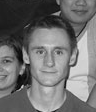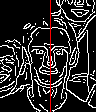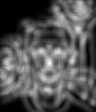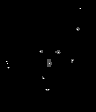Figure 7. From left to right: the input image group_shot_cropped (a photo), the lines of symmetry found (shown in red, superimposed on the edgel data), a plot of the objective function, and the objective function image after thresholding (grey pixels are non-maxima, white pixels are maxima).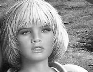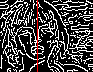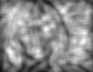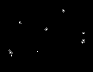Figure 8. The input image Venus (a painting), and output generated as shown in Figure 7.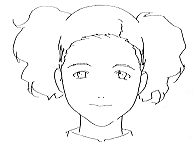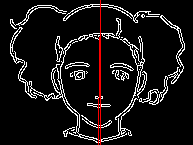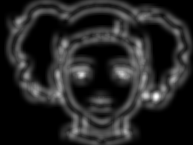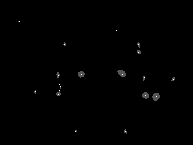Figure 9. The input image juri (a drawing), and output generated as shown in Figure 7.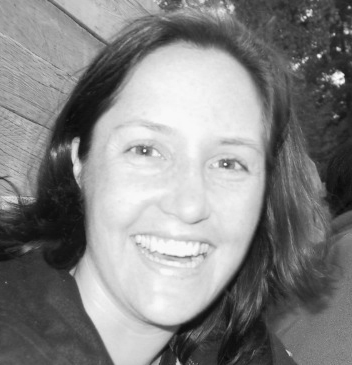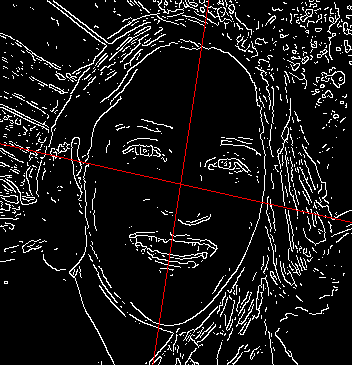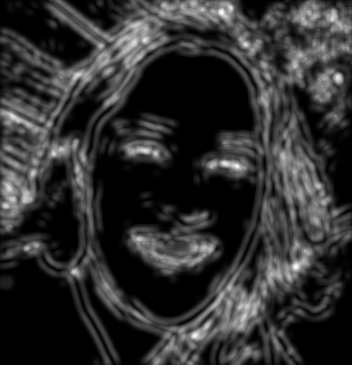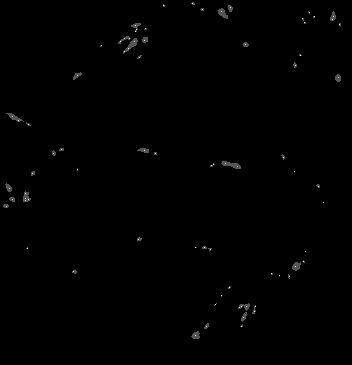Figure 10. The input image classy_kaye_cropped (a photo), and output generated as shown in Figure 7.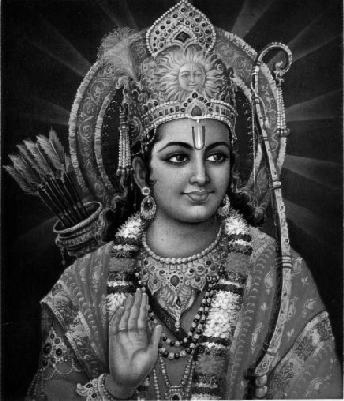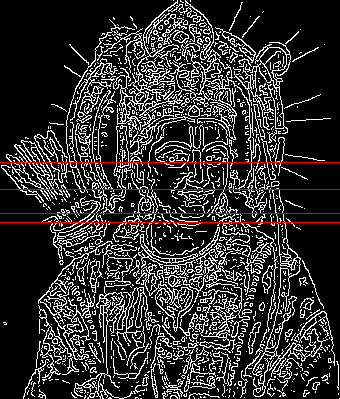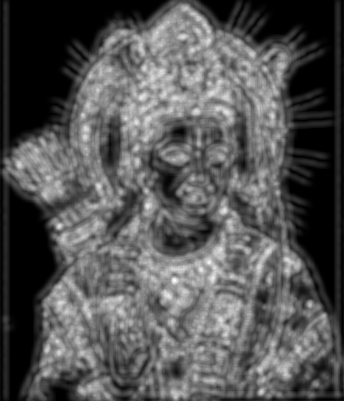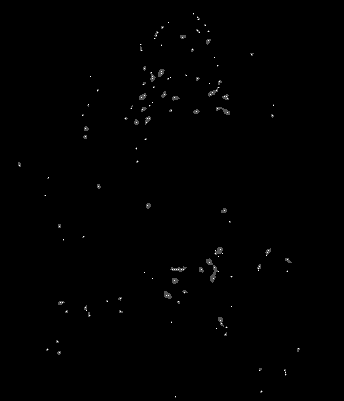Figure 11. The input image Rama (a painting), and output generated as shown in Figure 7.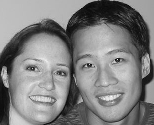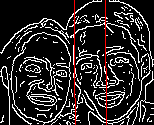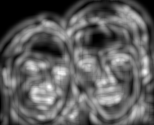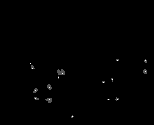Figure 12. The input image kayeandhilary (a photo), and output generated as shown in Figure 7.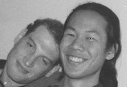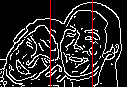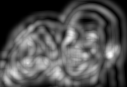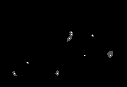Figure 13. The input image spring2k1_02 (a photo), and output generated as shown in Figure 7.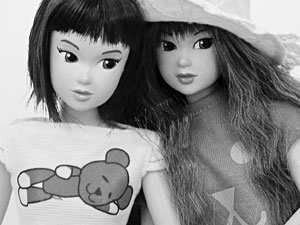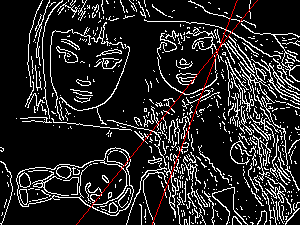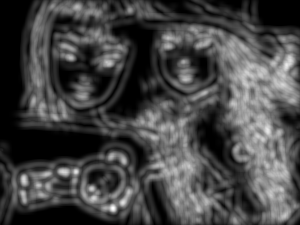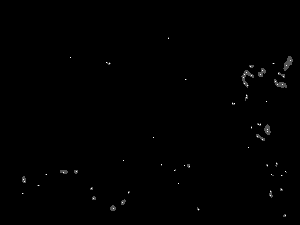Figure 14. The input image dhex (a photo of dolls), and output generated as shown in Figure 7.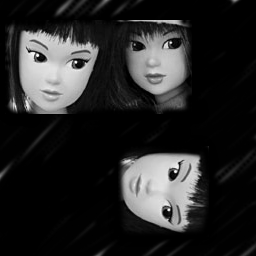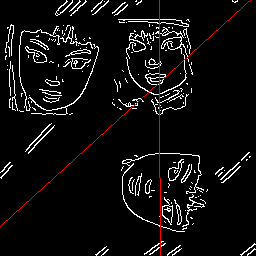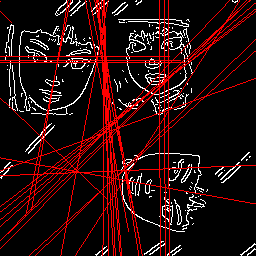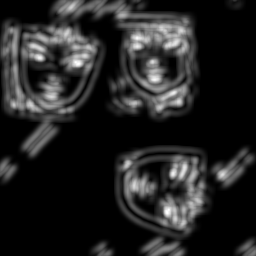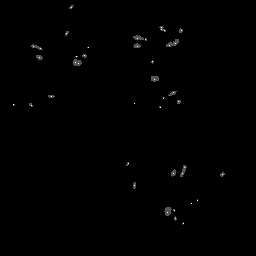Figure 15. From left to right: the input image dhex6 (a photo of dolls, after editing), the lines of symmetry found (shown in red, superimposed on the edgel data), the lines of symmetry found after reducing the threshold enough to find all three face's symmetries in the Hough transform (in total about 35 lines of symmetry pass the lowered threshold), a plot of the objective function, and maxima found in the objective function.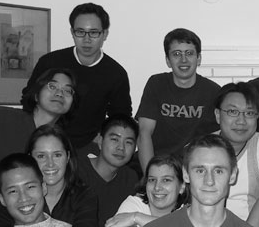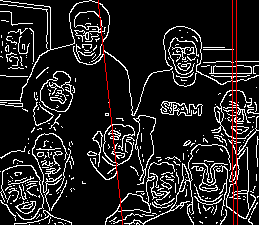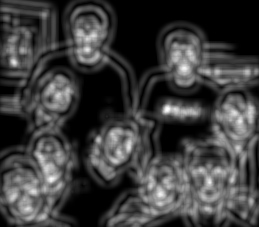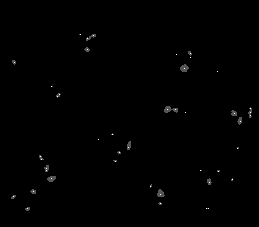Figure 16. The input image group_shot (a photo), and output generated as shown in Figure 7.

### Additional Results

In the course of testing the system with faces under different orientations, the situation in Figure 17 was encountered. Despite the lack of visual features on either side of the face, the system was unable to detect the face's line of symmetry. After some testing, the system was modified to highlight the edgels that contribute to a given candidate line of symmetry (Figure 17, right). As can be seen, we seem to have an unhappy accident, where many edgels happen to line up and contribute to the line of symmetry through the eyes.

The input image was subsequently rotated counterclockwise by a small angle to see if the phenomenon would persist (Figure 18). Indeed, it did not, and the system was now able to find the face's line of symmetry. However, from the highlighted edgels in Figure 18 we see that only a fraction of the face contributes to the detected line of symmetry.

Thus, we seem to have two potential problems. First, as shown in Figure 17, the systems appears to be sensitive to "accidental" symmetry. Second, as shown in Figure 18, even when the system correctly identifies the desired symmetry, it appears that only a fraction of the available image information is used, which means the system is fragile.

Examining the Hough transforms, we see that the bright spot corresponding to the face's line of symmetry is somewhat "smeared" out over many pixels. Since the search through the Hough transform only looks for maxima, most or all of the pixels over this smeared area are not considered. An M-estimation algorithm, appropriately initialized (e.g. using information from the Hough transform), would probably do a better job of "feeling out" the smeared area and making use of more of the edgel symmetries in the face.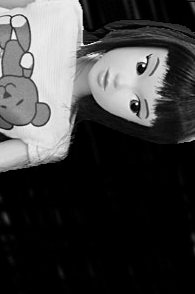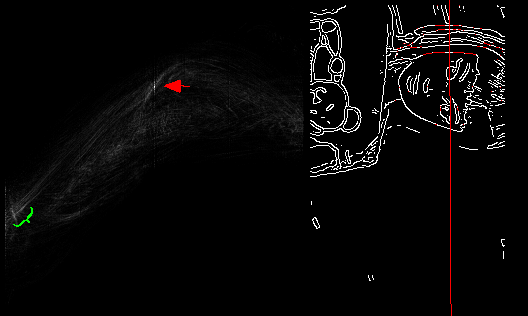Figure 17. From left to right: The input image dhex7 (an edited photo of a doll), the Hough transform, and the detected line of symmetry. The red arrow indicates the maximum in the Hough transform corresponding to the detected line of symmetry. The green brace shows the region of the Hough transform corresponding to the desired line of symmetry of the face.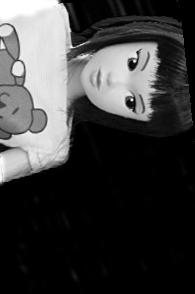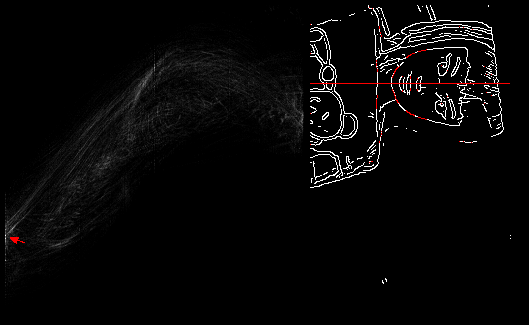Figure 18. From left to right: The input image dhex16 (a slightly rotated version of dhex7), the Hough transform, and the detected line of symmetry. The red arrow indicates the maximum in the Hough transform corresponding to the detected line of symmetry.

### Conclusions

There were many cases in which the symmetry detector did not succeed in finding the line(s) of symmetry of face(s) in the input: when there were many features in the environment surrounding the face (e.g. Figure 11), when there were multiple faces (e.g. Figure 12), when "accidental" or spurious symmetries were present (e.g. Figure 17). There were also cases, however, where the symmetry detector worked as desired.

To improve the performance of the detector, then, the input should preferably contain only one face, and contain little surrounding edgel information that could confuse the detector. The implementation of an M-estimation search through parameter space might also reduce the "distraction" due to accidental symmetries such as in Figure 17.

Because the symmetry detector performs a brute force check of every pair of edgels for cocircularity, its running time is quadratic in the number of pixels, which limits its use to lower resolution input (especially if real-time detection is required, as for a PUI).

In the test images presented, the loop detector seemed to perform well in many cases. Eyes in the input image were usually detected as loops. However, there are potentially many other non-eyes in the input that may be identified as loops (e.g. Figures 11 and 14). Hence, the loop detector alone would probably be of little use for locating eyes. Symmetry information, however, could be used to inform the detection of eyes.

### Future Directions

Following are lists of suggestions and ideas for tweaking, improving, or extending the detection algorithms.

Improving Symmetry Detection

Suggestions for reducing the number of pairs of edgels to be considered (and also potentially enhance the Hough transform, making it easier to find maxima):

• Experiment with reducing thetaepsilon
• Experiment with different values for Dmin and Dmax (for example, the value of Dmax could be based on the maximum expected size of a face)
• Only consider axes of symmetry that are approximately vertical
• All of the preceding suggestions would only reduce running time by a constant amount. The number of pairs of edgels would still be quadratic in the number of pixels. However, if the symmetry detector were applied to multiresolution representations of the input, running time could be decreased more significantly. For example, a search for symmetry could be made at a high-level (by first downsampling the input image to be of some fixed, low resolution) and also at a low level (by dividing the full resolution input into subimages that are each 64x64 pixels, and checking for symmetry within each of these). If symmetry is detected at either the high or low level, the algorithm could then work its way down or up appropriately. Note that this runs the risk of missing features that are only symmetric at a mid-level, but this is an acceptable risk under appropriate assumptions (e.g. if the range of sizes of faces is known).

Potential ways to enhance the Hough transform, to make it easier to find maxima:

• Candidate lines of symmetry could be weighted by how close the corresponding edgels are to each other (so pairs of edgels that are cocircular and proximal would be weighted more -- e.g., the nose and mouth in a face would contribute more strongly that the ears or hair, which are less likely to be perfectly symmetric anyways).
• Candidate lines of symmetry could be weighted by how small the mismatch is between reflected edgel orientations (so pairs of edgels that are very exactly cocircular would be weighted more).
• Coincident points in the Hough transform (i.e. multiple instances of the same candidate line of symmetry) could be more heavily weighted if the corresponding edgels are proximal in image space (so sets of symmetric edgels are weighted more if they are connected together).

Improving Eye Detection

Alternative strategies for detecting loops:

• A strategy similar to the one already presented (i.e. computing an objective function from the edgels and searching for maxima) could be used, however, rather than having each edgel contribute a component to the objective function, we could have each pair of neighbouring edgels contribute a component on the concave side of the edgels. Thus, long curved chains of edgels would result in a large contribution in the region cupped by the chain. The distance of the contribution from each pair of edgels could be computed based on the angle between the pair, which would eliminate the need for a built-in expected eye size reye.
• Fitting elliptic segments  to the edgel data to try and find loops or parts of loops.
• Using snakes  to try and find loops of edgels. Note that snakes often don't converge well if they are not initialized near the target, so it is perhaps dubious whether they would be workable.
• Searching for chains of cocircular edgels. Note that the loops around eyes are often not strictly closed or even connected, so there would be many problems with this technique.

Alternative strategies for detecting eyes:

• Using eigen eyes. Note that, unless the expected size of the eyes is known, an eigen eye detector would have to be applied at multiple different resolutions of the input image.

Building a Face Detector

Finding the major axis of the face and the location of the eyes may be all that is needed to build an interesting PUI. However, the loop detector presented here often detects many more loops than just those corresponding to eyes. We need some way of eliminating the non-eyes.

Assuming we have correctly identified the line of symmetry L of the face, we can then search through the loops found in the image for two loops that approximately reflect onto each other through L. To do this, we could (for example) compute the distance di between each loop i and L, and also the point pi resulting from projecting i onto L. We could then search for the pair of loops for which the difference between their di and pi is a minimum.

As a refinement to this strategy, we could first take all edgels that contributed to L, project them onto L, and compute a minimal bounding line segment L' covering all such projections. L' would presumably be an approximation of the line segment connecting the face's chin to the top of the forehead. Then, when we search for loops that represent eyes, we could constrain the search to loops whose pi falls on L'. Any other loops would presumably not be part of the face. We could also compute a threshold for the expected maximum difference between the di and pi of the eyes -- computing the threshold based on the length of L'.

Unfortunately, we may not succeed in identifying the correct line of symmetry for the face, or we may detect multiple possible lines of symmetry. A more robust system would probably use information from both the symmetry detection and loop detection stages to inform and narrow the selection of potential lines of symmetry and loops that represent eyes.

### Acknowledgements

The author is grateful to Allan Jepson and Chakra Chennubhotla for their guidance and thoughtful comments.

### References

1. Black, M. J. and Yacoob, Y. Tracking and recognizing rigid and non-rigid facial motions using local parametric models of image motion, Fifth International Conf. on Computer Vision, ICCV'95, Boston, MA, June 1995, pp 374-381.
2. Black, M. J. and Yacoob, Y. Recognizing facial expressions under rigid and non-rigid facial motions using local parametric models of image motion, International Workshop on Automatic Face- and Gesture-Recognition, Zurich, July 1995.
3. Black, M. J., Yacoob, Y., Jepson, A. D., Fleet, D. J., Learning parameterized models of image motion, IEEE Conf. on Computer Vision and Pattern Recognition, CVPR-97, Puerto Rico, June 1997, pp. 561-567.
4. Paul Bourke. PPM / PGM / PBM image files, July 1997. http://astronomy.swin.edu.au/~pbourke/dataformats/ppm/
5. Cootes and Taylor. Statistical models of appearance for medical image analysis and computer vision. Proc. SPIE Medical Imaging, 2001. http://www.isbe.man.ac.uk/~bim/refs.html
6. Herpers R., H. Kattner, H. Rodax and G. Sommer. GAZE: An Attentive Processing Strategy to Detect and Analyze the Prominent Facial Regions. Proc. of Int. Workshop on Automatic Face- and Gesture-Recognition, IWAFGR'95, M. Bichsel (edt.), Zurich, Switzerland, pp. 214-220, 1995.
7. Matlab edgel detection routines. Source code available as part of Assignment 2, CSC 487/2503, Fall 2001, University of Toronto. http://www.cs.toronto.edu/~jepson/csc2503/
8. Trucco and Verri. Introductory Techniques for 3-D Computer Vision. Prentice Hall, 1st edition, 1998. ISBN: 0132611082.